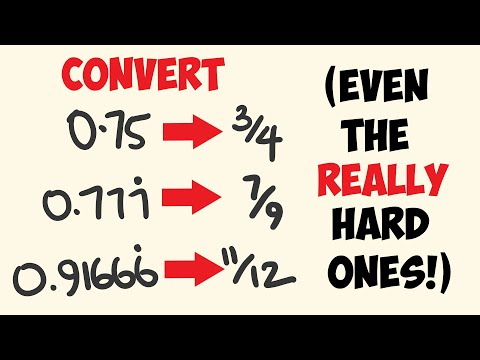Home » What is 2 as a Fraction? – Decimal to Fraction Conversion

# What is 2 as a Fraction? – Decimal to Fraction Conversion

What is 2 as a Fraction?

• A. 5/8
• B. 2/1
• C. 1/2
• D. 3/5
• E. 129/100

## Solution:

As a fraction, the number 2 can be written as 2/1. This is because you get the same value if you divide any number by 1. So, if you divide 2 by 1, you get 2.

If the numerator and denominator are the same, the fraction equals 1. The fraction is more than 1 in this case because the numerator is more significant than the denominator. We call this an “improper fraction.”

So, when 2 is written as a fraction, it is written as 2/1.

## Conceptual Understanding of Fractions

Fractions are used to show parts of a whole. The top part of the fraction, called the numerator, shows how many parts we have, and the bottom part, called the denominator, shows how many equal parts the whole is divided into. In the end, a fraction is just a problem with division. You can think of a fraction as dividing the numerator by the denominator.

## Conversion of Whole Numbers to Fractions

Converting whole numbers into fractions is a straightforward process. You can express any whole number as a fraction by placing it over 1. The reason for this is straightforward: dividing a whole number by 1 yields the original whole number. In other words, when something is divided into one portion, that portion is equivalent to the original whole.

## The Fractional Representation of 2

To express 2 as a fraction, we simply place it over 1, resulting in the fraction 2/1. This fraction indicates that there are two of the same unit. The result of dividing 2 ÷ 1 equals 2, proving that 2/1 is equivalent to the whole number 2. 2/1 is the simplest fractional representation of the whole number 2.